# 算法,永远滴神之【链表结构】

### 一、常见数据结构

#### 针对数组是否是线性表的争议？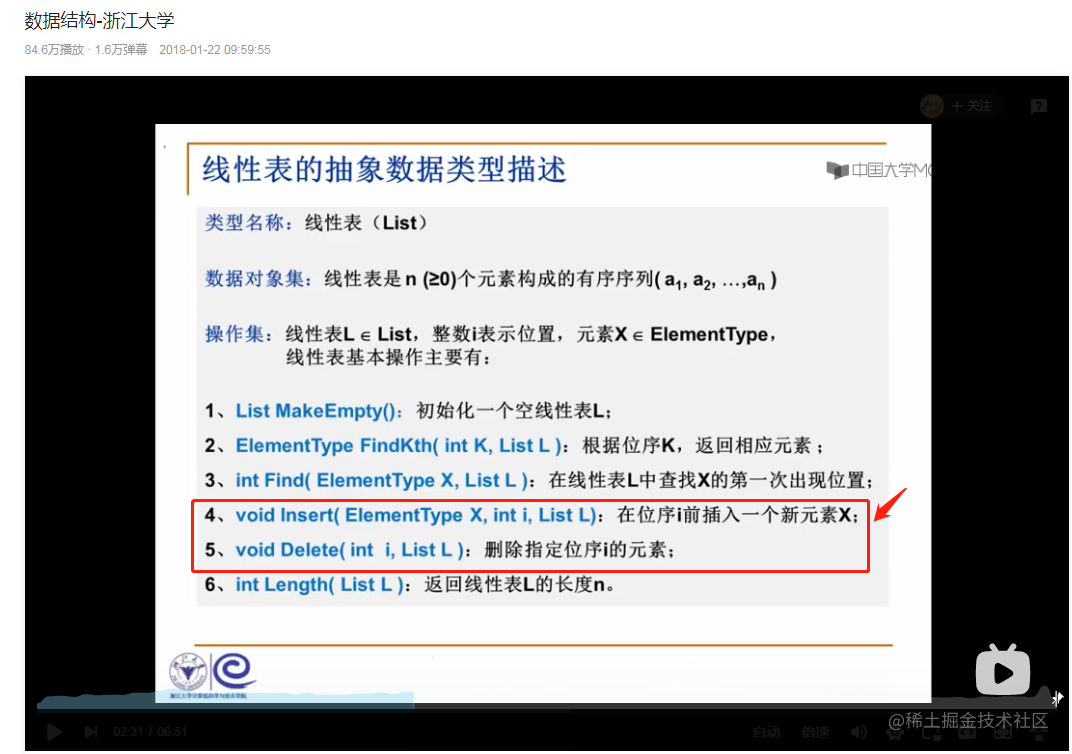### 二、链表

#### 2.1 顺序链表

1.java里面的顺序列表，代表为ArrayList。里面实现是利用（对象）数组实现，扩容是利用算法计算的，如果超出当前数组长度 就会使用新数组，然后把原值针对下标赋值给新数组，最后把指针指向原对象数组。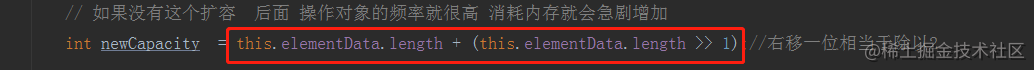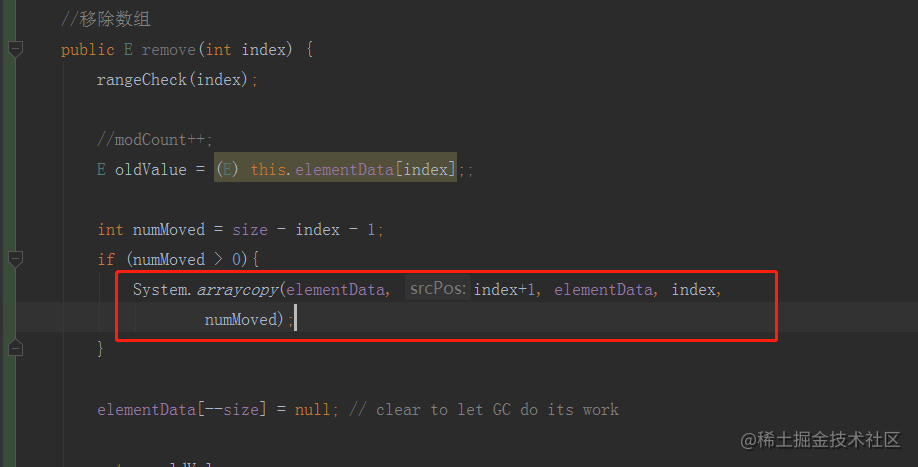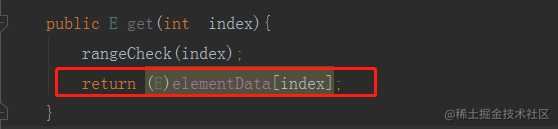##### 总结

``````import java.util.*;
/**
*  <li>@author: 程序员ken </li>
* <li>Description: 顺序表 </li>
* </ul>
*/
public class MyList<E> {

private static final int DEFAULT_CAPACITY = 10;

private static final Object[] DEFAULTCAPACITY_EMPTY_ELEMENTDATA = {};

transient Object[] elementData;

//数组长度
private  int size = 0;

private static final int MAX_ARRAY_SIZE = Integer.MAX_VALUE - 8;

public MyList() {
this.elementData = DEFAULTCAPACITY_EMPTY_ELEMENTDATA;
}

if(this.elementData == DEFAULTCAPACITY_EMPTY_ELEMENTDATA){
this.elementData = new Object[DEFAULT_CAPACITY];
}
//当前新增 超出元素个数 (需要扩容)
if(size+1>this.elementData.length){
// 超出元素 每次扩容10
//Object[] objects = new Object[DEFAULT_CAPACITY + this.elementData.length];

// 如果没有这个扩容  后面 操作对象的频率就很高 消耗内存就会急剧增加
int newCapacity  = this.elementData.length + (this.elementData.length >> 1);//右移一位相当于除以2

if(MAX_ARRAY_SIZE < newCapacity){
throw new RuntimeException("超出容器最大容量");
}

else if(size+1>newCapacity){
newCapacity = size+1;
}

//            if(MAX_ARRAY_SIZE< DEFAULT_CAPACITY + this.elementData.length){
//                throw new RuntimeException("超出容器最大容量");
//            }
//           for (int i = 0; i <this.elementData.length ; i++) {
//                objects [i] = this.elementData[i];
//            }
//            this.elementData = objects;

this.elementData =  Arrays.copyOf(this.elementData, newCapacity);
}

}
this.elementData[size++]=e;
}

//移除数组
public E remove(int index) {
rangeCheck(index);

//modCount++;
E oldValue = (E) this.elementData[index];;

int numMoved = size - index - 1;
if (numMoved > 0){
System.arraycopy(elementData, index+1, elementData, index,
numMoved);
}

elementData[--size] = null; // clear to let GC do its work

return oldValue;
}

public E get(int  index){
rangeCheck(index);
return (E)elementData[index];
}

//范围检查
private void rangeCheck(int index) {
if (index >= size){
throw new IndexOutOfBoundsException(String.format("Index:%s, Size:%s",index,this.size));
}
}

private int size() {
return  this.size;
}

}

#### 2.1 单链表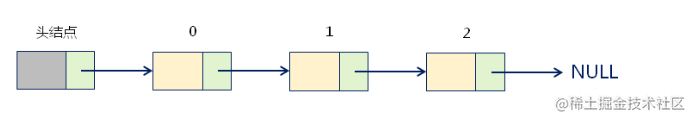##### 特点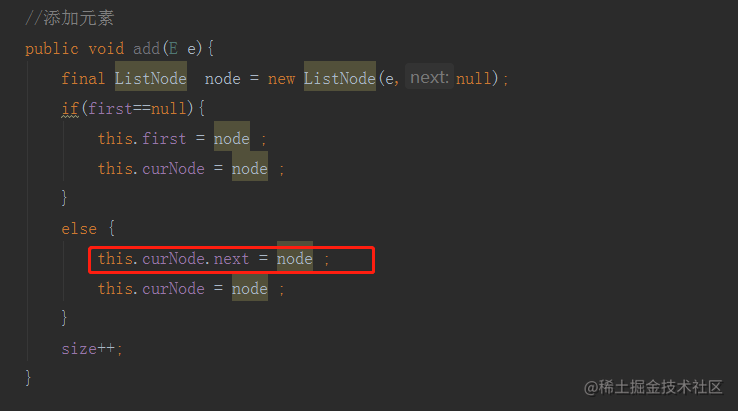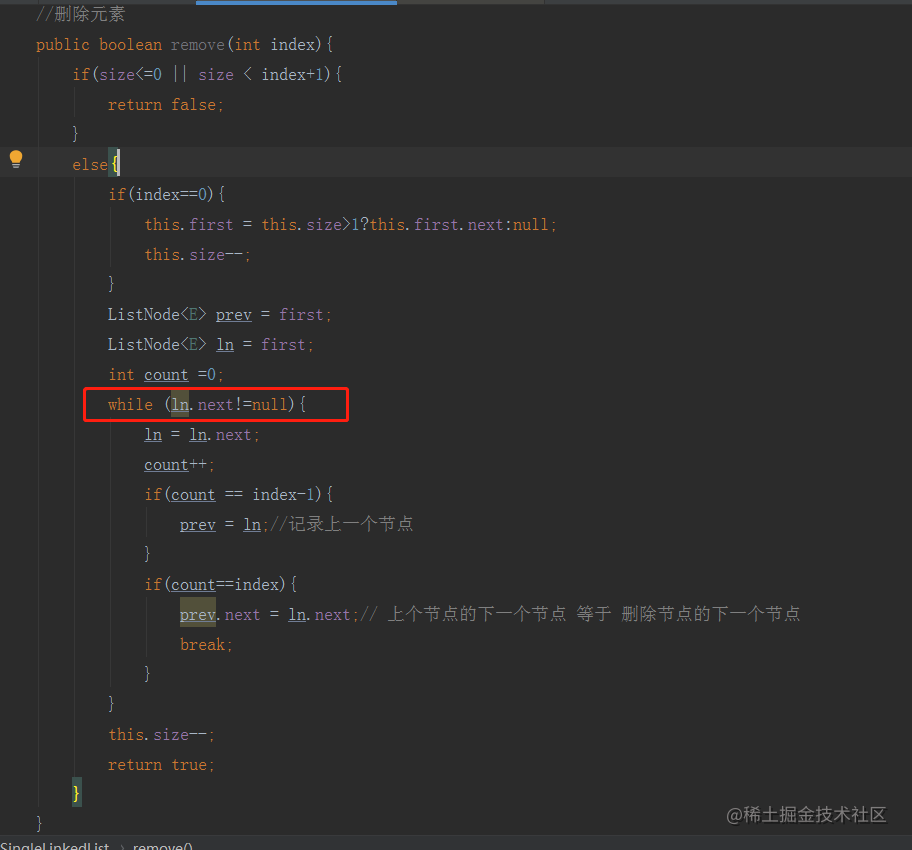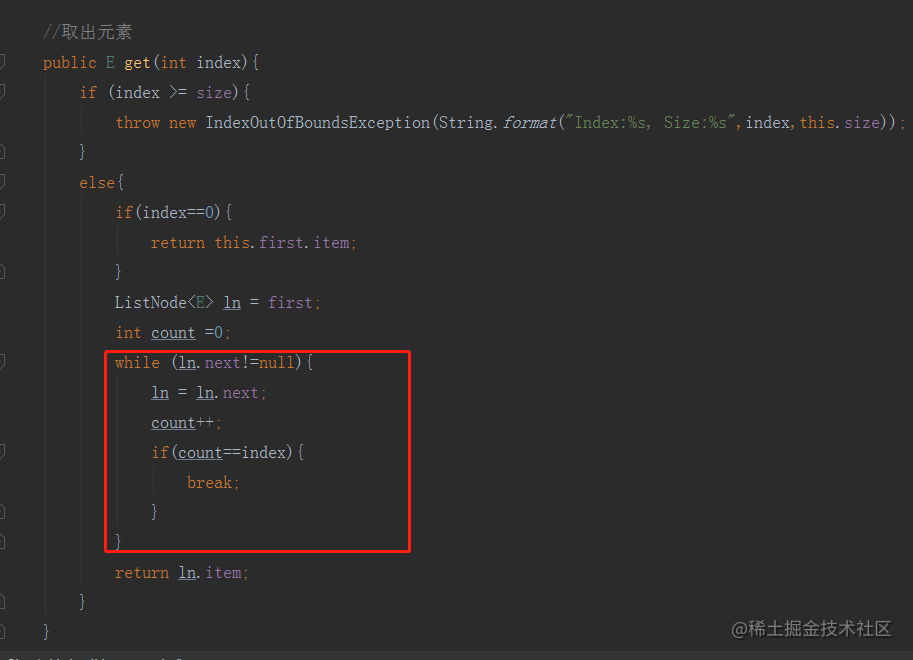##### 总结

``````

/**
* <ul>
* <li>Description: 单链表 </li>
* </ul>
*
* @author 程序员ken
* @date 2021/5/21 0021 上午 9:59
*/
transient ListNode<E> first; // 头部节点
transient ListNode<E> curNode;//当前操作节点（因为找不到前置节点，所以需要记录前置节点）
private  int size;

//添加元素
final ListNode  node = new ListNode(e,null);
if(first==null){
this.first = node ;
this.curNode = node ;
}
else {
this.curNode.next = node ;
this.curNode = node ;
}
size++;
}

//删除元素
public boolean remove(int index){
if(size<=0 || size < index+1){
return false;
}
else{
if(index==0){
this.first = this.size>1?this.first.next:null;
this.size--;
}
ListNode<E> prev = first;
ListNode<E> ln = first;
int count =0;
while (ln.next!=null){
ln = ln.next;
count++;
if(count == index-1){
prev = ln;//记录上一个节点
}
if(count==index){
prev.next = ln.next;// 上个节点的下一个节点 等于 删除节点的下一个节点
break;
}
}
this.size--;
return true;
}
}

//取出元素
public E get(int index){
if (index >= size){
throw new IndexOutOfBoundsException(String.format("Index:%s, Size:%s",index,this.size));
}
else{
if(index==0){
return this.first.item;
}
ListNode<E> ln = first;
int count =0;
while (ln.next!=null){
ln = ln.next;
count++;
if(count==index){
break;
}
}
return ln.item;
}
}

public int size(){
return this.size;
}

// 定义单链表结构
static class ListNode<E> {
private E item;
private ListNode<E> next;

public ListNode(E item, ListNode<E> next) {
this.item = item;
this.next = next;
}
}

}

#### 2.1 双（向）链表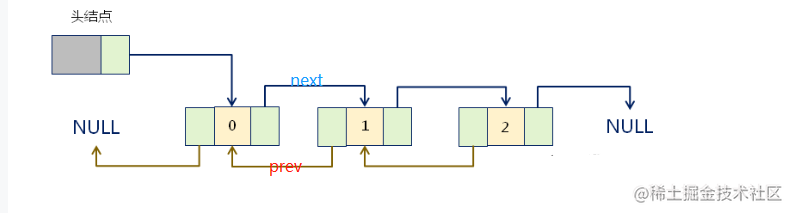##### 特点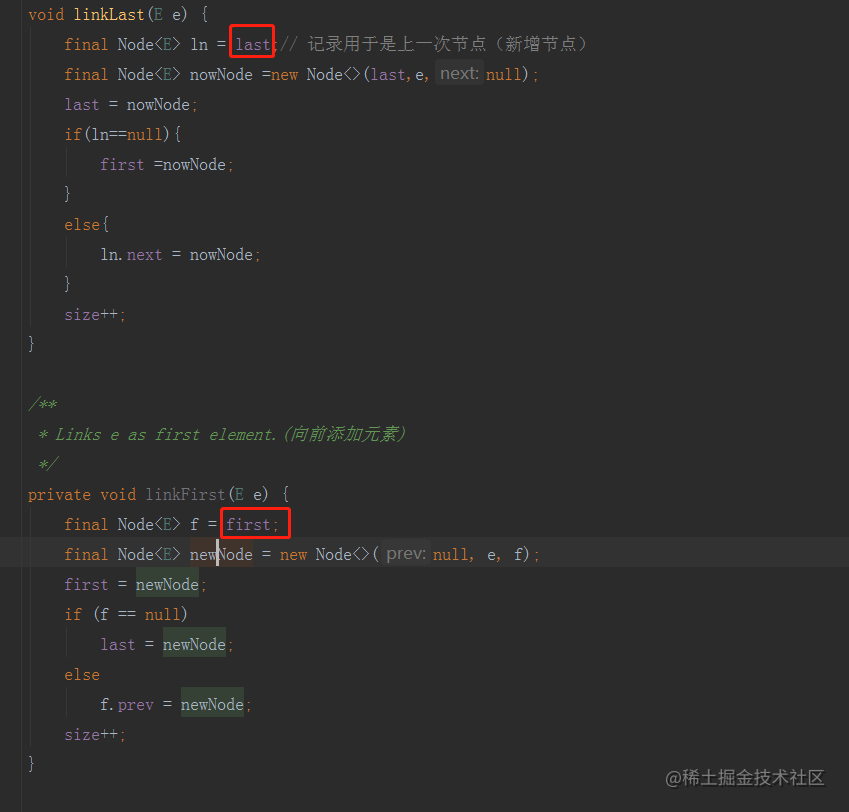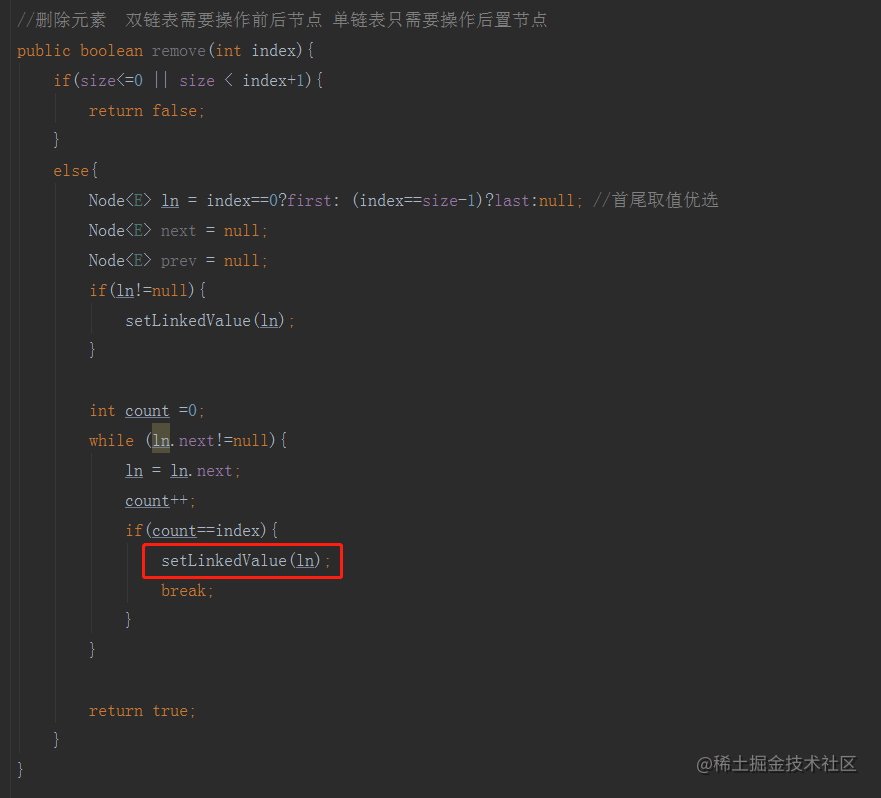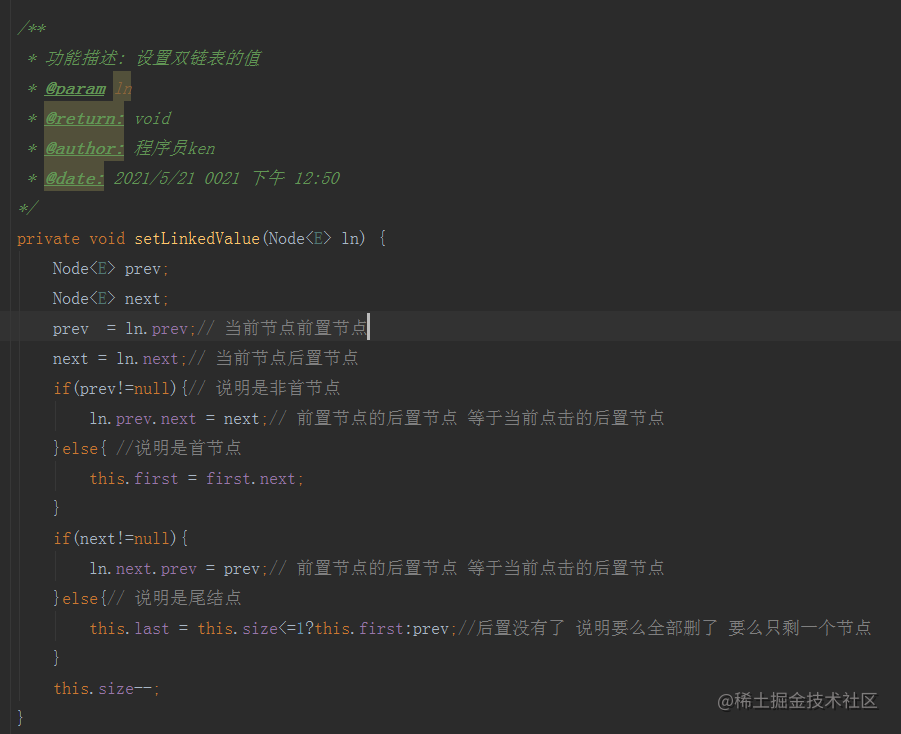##### 总结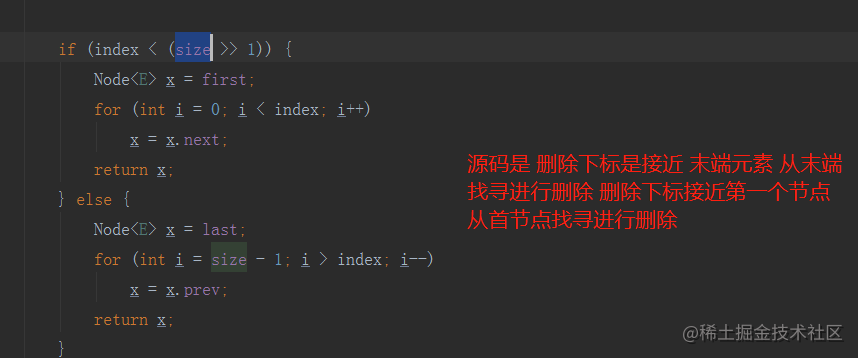（这个是源码里面的，我手写的是从首节点向下寻找删除节点的）

``````

/**
* <ul>
* <li>Description: 双向链表 </li>
* </ul>
*
* @author 程序员ken
* @date 2021/5/20 22:16
*/

private int size = 0;

transient Node<E> first;

transient Node<E> last;

return true;
}

/**
* Links e as last element.(向后添加元素)
*/
final Node<E> ln = last;// 记录用于是上一次节点（新增节点）
final Node<E> nowNode =new Node<>(last,e,null);
last = nowNode;
if(ln==null){
first =nowNode;
}
else{
ln.next = nowNode;
}
size++;
}

/**
* Links e as first element.(向前添加元素)
*/
final Node<E> f = first;
final Node<E> newNode = new Node<>(null, e, f);
first = newNode;
if (f == null)
last = newNode;
else
f.prev = newNode;
size++;
}

//删除元素  双链表需要操作前后节点 单链表只需要操作后置节点
public boolean remove(int index){
if(size<=0 || size < index+1){
return false;
}
else{
Node<E> ln = index==0?first: (index==size-1)?last:null; //首尾取值优选
Node<E> next = null;
Node<E> prev = null;
if(ln!=null){
}

int count =0;
while (ln.next!=null){
ln = ln.next;
count++;
if(count==index){
break;
}
}

return true;
}
}

/**
* 功能描述: 设置双链表的值
* @param ln
* @return: void
* @author: 程序员ken
* @date: 2021/5/21 0021 下午 12:50
*/
Node<E> prev;
Node<E> next;
prev  = ln.prev;// 当前节点前置节点
next = ln.next;// 当前节点后置节点
if(prev!=null){// 说明是非首节点
ln.prev.next = next;//前置节点的后置节点 等于当前节点的后置节点
}else{ //说明是首节点
this.first = first.next;
}
if(next!=null){
ln.next.prev = prev;// 前置节点的后置节点 等于当前节点的后置节点
}else{// 说明是尾结点
this.last = this.size<=1?this.first:prev;//后置没有了 说明要么全部删了 要么只剩一个节点
}
this.size--;
}

//取出元素
public E get(int index){
if (index >= size){
throw new IndexOutOfBoundsException(String.format("Index:%s, Size:%s",index,this.size));
}
else{
//首尾取值优选
if(index==0 || index== this.size-1){
return index == this.size-1?this.last.item:this.first.item;
}
Node<E> ln = first;
int count =0;
while (ln.next!=null){
ln = ln.next;
count++;
if(count==index){
break;
}
}
return ln.item;
}
}

public int size(){
return this.size;
}

static class Node<E>{
E item;
Node<E> next;
Node<E> prev;

Node(Node<E> prev, E element, Node<E> next) {
this.item = element;
this.next = next;
this.prev = prev;
}

}

}

### 三、关于链表的算法题

【题目描述】：

4

1 2 3 4

5

1 2 3 5 6

**上面链表1的内容为【1, 2 ,3 ,4】,链表1的内容为【1, 2 , 3 , 5, 6】，记住链表元素是单调递增的。公共部分为【1,2,3】 **